Question

A Semi-infinite beam is loaded by force P at distance a from its end, as shown in figure. (a) Obtain formulas for deflection, slope, moment, and shear force, Using the results from part (a), consider a 2-m-long steel bar (E = 210 GPa) of 75mm´75mm square cross section that rests with a side on a rubber foundation with a modulus of k = 24 MPa. If a concentrated load P = 100 kN is applied at the distance of a = 0.30 m, (b) Calculate Maximum bending stress inside the beam, (c) Calculate the maximum deflection and the maximum force per unit length between beam and foundation.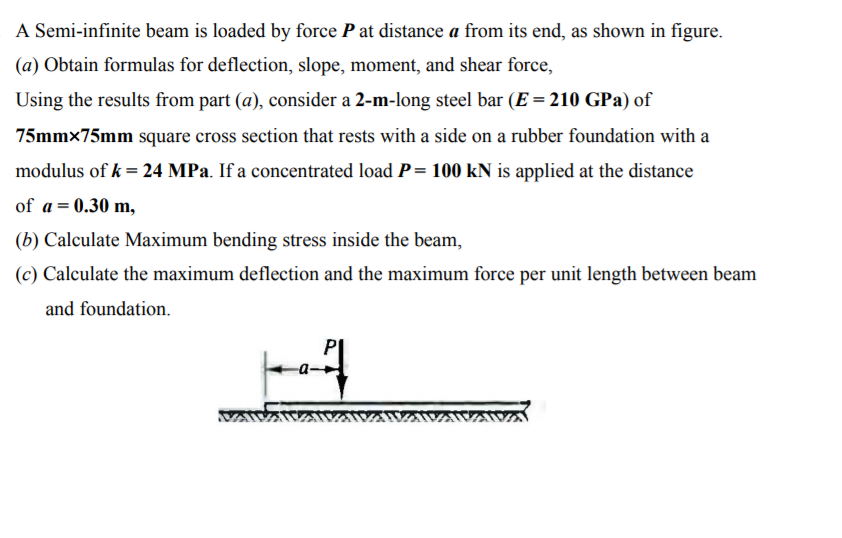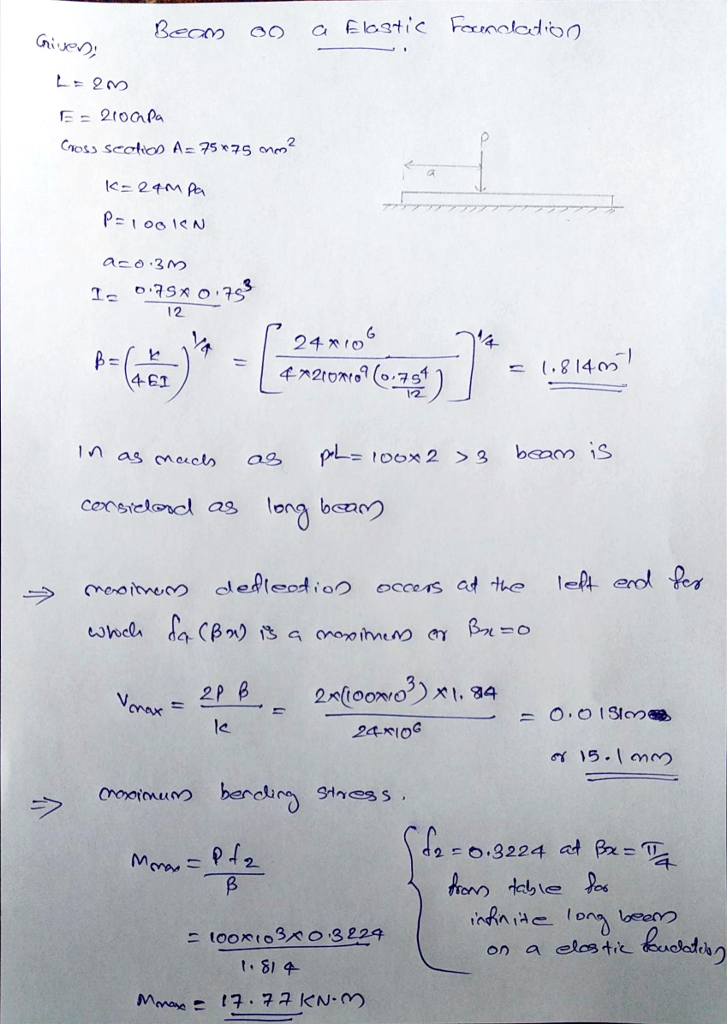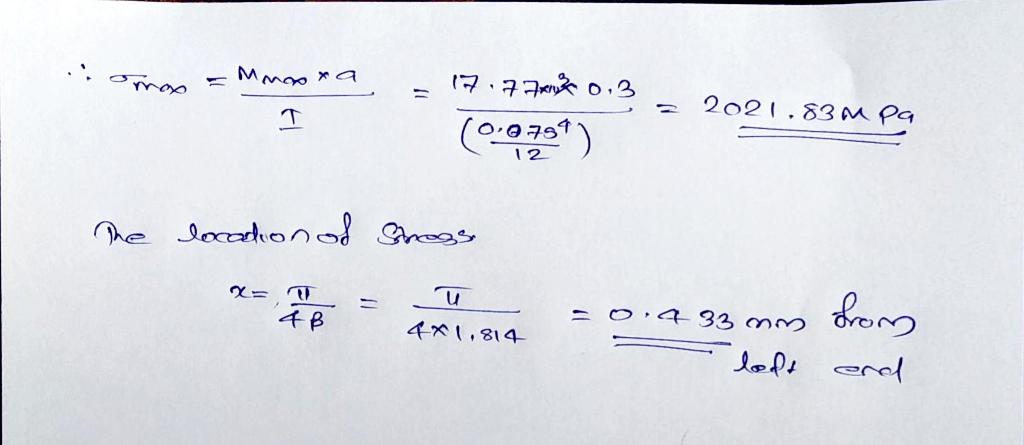#### Earn Coins

Coins can be redeemed for fabulous gifts.

Similar Homework Help Questions
• ### The beam is loaded as shown in the diagram below. The beam is uniformly loaded at...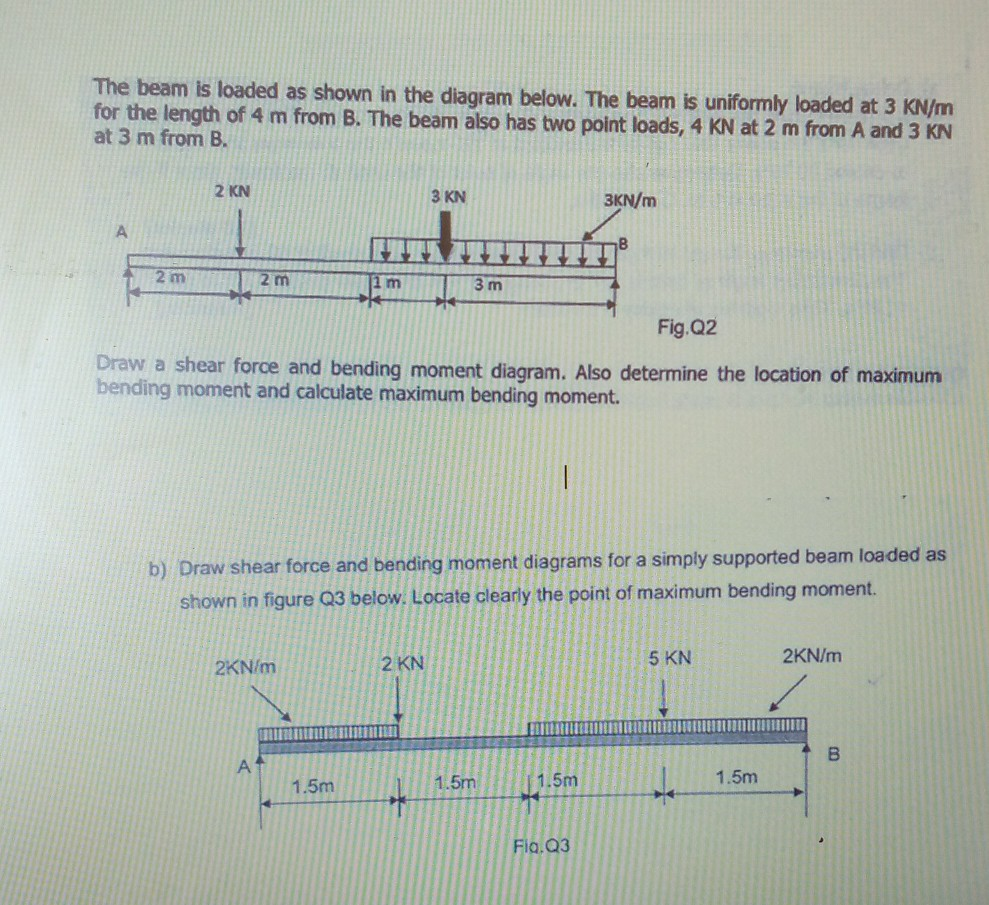The beam is loaded as shown in the diagram below. The beam is uniformly loaded at 3 kN/m for the length of 4 m from B. The beam also has two point loads, 4 KN at 2 m from A and 3 KN at 3 m from B. 2 KN 3 KN 3KN/m A 2 m 2 m 11 m 3 m Fig. Q2 Draw a shear force and bending moment diagram. Also determine the location of maximum bending moment...

• ### A simply support beam is loaded as shown in the following figure. 1) Draw the shear...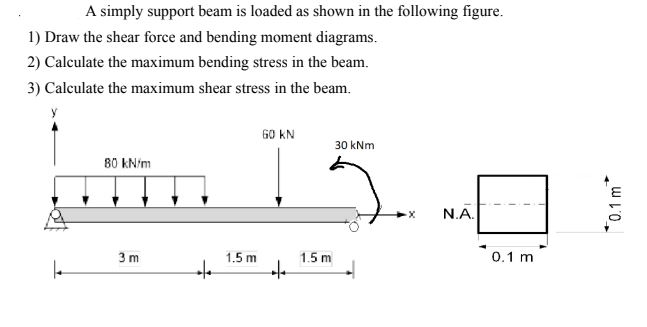A simply support beam is loaded as shown in the following figure. 1) Draw the shear force and bending moment diagrams. 2) Calculate the maximum bending stress in the beam. 3) Calculate the maximum shear stress in the beam. G0 KN 30 kNm B0 kN/m H NA 0.1 m 3 m e 1.5m to 1.5m. 0.1 m

• ### 2. A cantilever beam is loaded as shown in the following figure. 1) Draw the shear...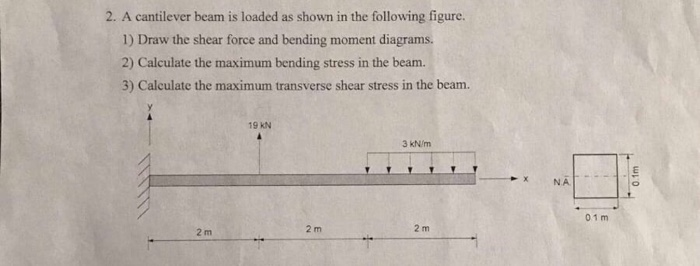2. A cantilever beam is loaded as shown in the following figure. 1) Draw the shear force and bending moment diagrams 2) Calculate the maximum bending stress in the beam. S 3) Calculate themaximum transverse shear stress in the beam. 19 kN 3 kN/m NA 01 m 2 m 2 m 2 m

• ### PLEASE PROVIDE THE SLOPE AND DEFELCTION DIAGRAM!!!!!!!!!!!!!! 7) A beam is supported and loaded as shown...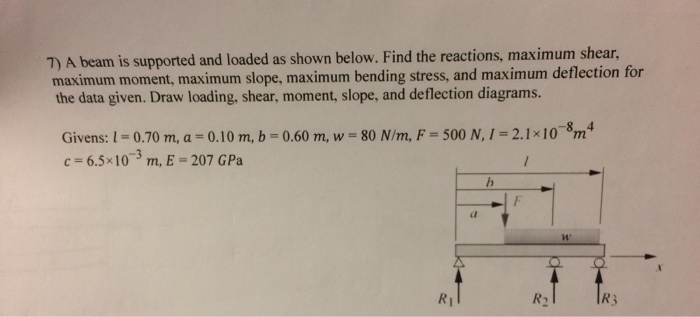PLEASE PROVIDE THE SLOPE AND DEFELCTION DIAGRAM!!!!!!!!!!!!!! 7) A beam is supported and loaded as shown below. Find the reactions, maximum shear, maximum moment, maximum slope, maximum bending stress, and maximum deflection for the data given. Draw loading, shear, moment, slope, and deflection diagrams. -8 4 Givens: 1 0.70 m, a = 0.10 m, b 0.60 m, w 80 N/m, F = 500 N,1 = 2.1x10m c 6.5x10 m, E = 207 GPa / R3 R1 R2 7) A beam...

• ### 7) A beam is supported and loaded as shown below. Find the reactions, maximum shear, maximum...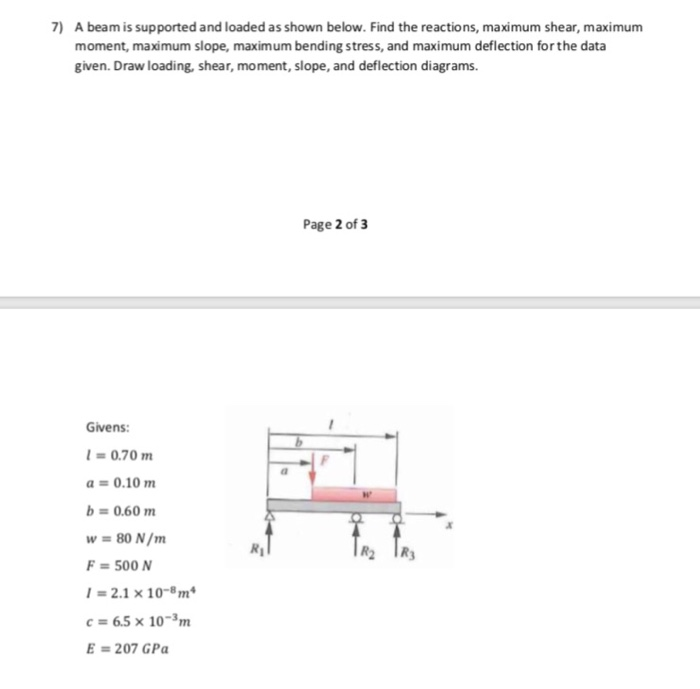7) A beam is supported and loaded as shown below. Find the reactions, maximum shear, maximum moment, maximum slope, maximum bending stress, and maximum deflection for the data given. Draw loading, shear, moment, slope, and deflection diagrams. Page 2 of 3 Givens: 0.70 m a 0.10 m b 0.60 m w 80 N/m F 500 N 2.1 x 10- m c 6.5 x 10-3m E=207 GPa 7) A beam is supported and loaded as shown below. Find the reactions, maximum...

• ### Strength of Materials IV 9.2-5 The defiuction curve for a cantilever beam AB (see fgure) is given b 120LEI Describe the load acting on the beam. 2 .3-6 Calculate the maximum deflectio...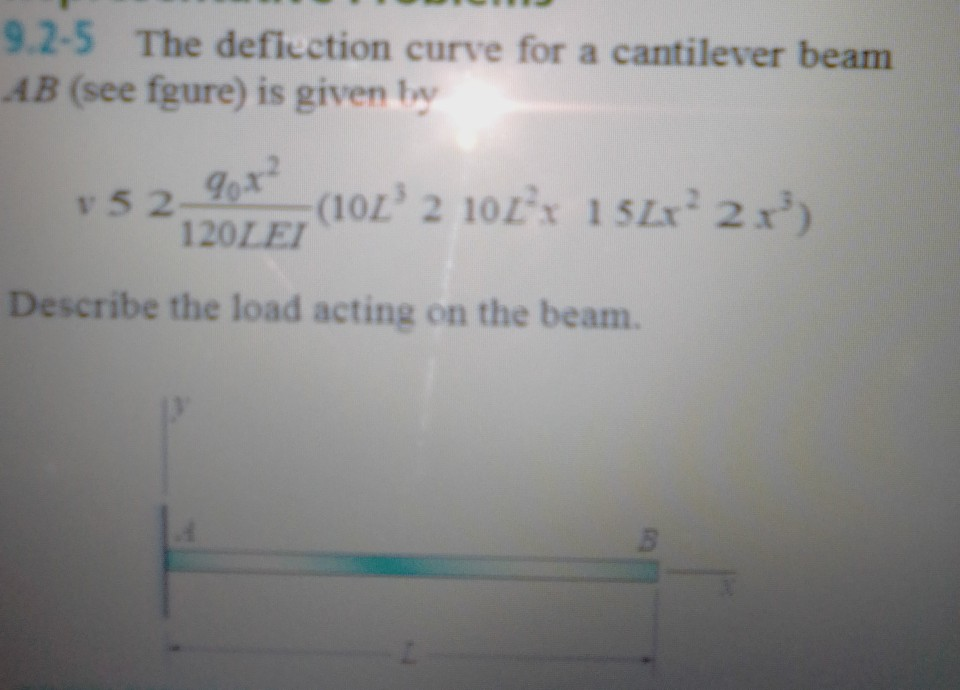Strength of Materials IV 9.2-5 The defiuction curve for a cantilever beam AB (see fgure) is given b 120LEI Describe the load acting on the beam. 2 .3-6 Calculate the maximum deflection dma of a uniformly loaded simple beam if the span length L 5 2.0 m, the intensity of the uniform load g 5 2.0 kN/m, and the maximum bending stress s 5 60 MPa. rn X The cross section of the beam is square, and the material is...

• ### A rectangular cross section at a location along a beam in bending is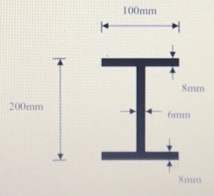(a). A rectangular cross section at a location along a beam in bending is acted upon by a bending moment and a shear force. The cross section is $$120 \mathrm{~mm}$$ wide, $$300 \mathrm{~mm}$$ deep and is orientated such that it is in bending about its major axis of bending. The magnitudes of the bending moment and shear force are $$315 \mathrm{kNm}$$ and $$240 \mathrm{kN}$$ respectively. Determine the maximum bending and shear stresses on the cross section. Plot the bending and...

• ### P=10 kN A cantilever beam is subiected to a concentrated force P, a uniformly distributed load...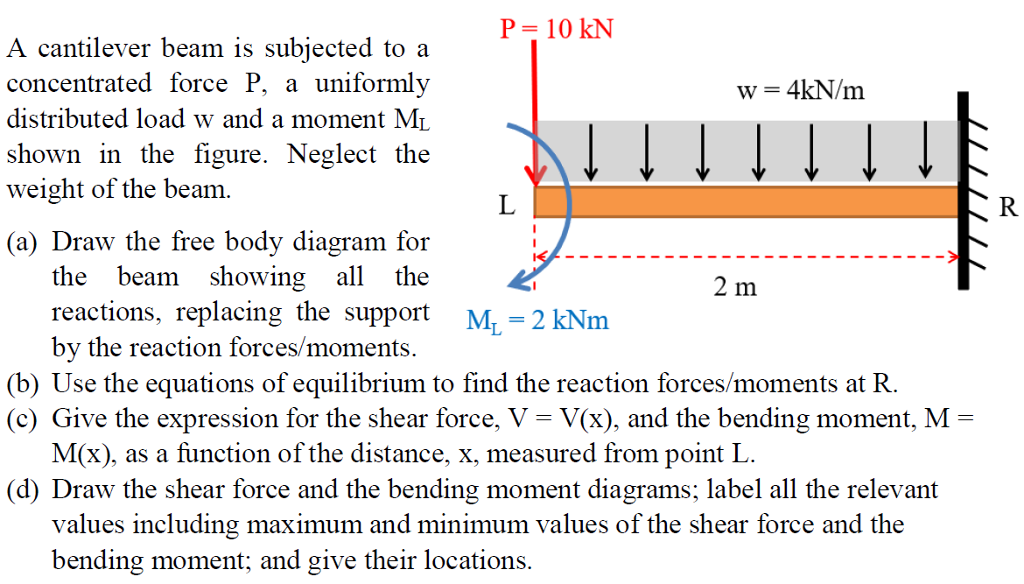P=10 kN A cantilever beam is subiected to a concentrated force P, a uniformly distributed load w and a moment MI shown in the figure. Neglect the weight of the beam. (a) Draw the free body diagram for the beam showing all the 2 m reactions, replacing the support M.-2 kNm by the reaction forces/moments. (b) Use the equations of equilibrium to find the reaction forces/moments at R (c) Give the expression for the shear force, V- V(x), and the...

• ### 4. A T-shaped cross-sectional beam is loaded as shown in the figure. Determine the following a....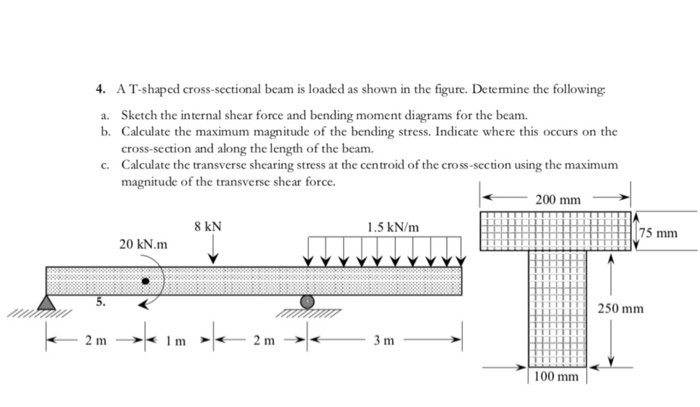4. A T-shaped cross-sectional beam is loaded as shown in the figure. Determine the following a. Sketch the internal shear force and bending moment diagrams for the beam. b. Calculate the maximum magnitude of the bending stress. Indicate where this occurs on the cross-section and along the length of the beam. c. Calculate the transverse shearing stress at the centroid of the cross-section using the maximum magnitude of the transverse shear force. - 200 mm 8 KN 1.5 kN/m 20...

• ### 20 KN 20 kN/m From the figure load given, the beam AB is I profile (S...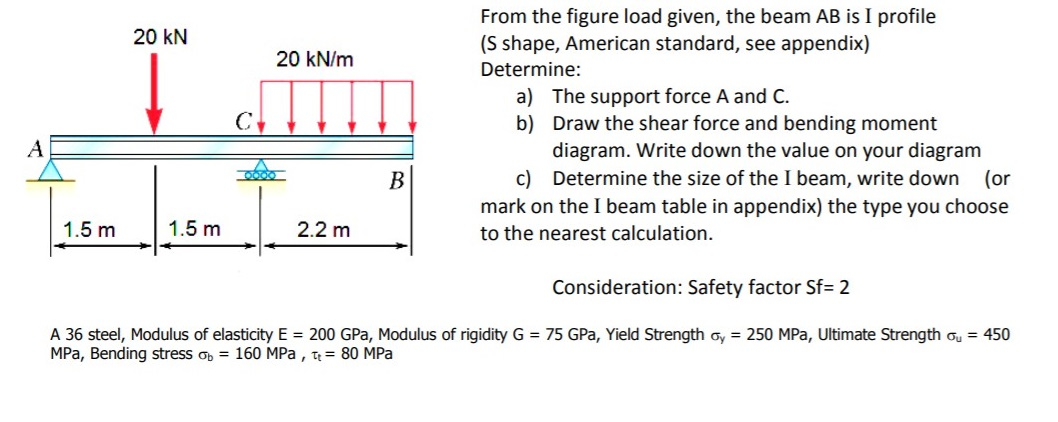20 KN 20 kN/m From the figure load given, the beam AB is I profile (S shape, American standard, see appendix) Determine: a) The support force A and C. b) Draw the shear force and bending moment diagram. Write down the value on your diagram c) Determine the size of the I beam, write down (or mark on the I beam table in appendix) the type you choose to the nearest calculation. A B 1.5 m 1.5 m 2.2 m...